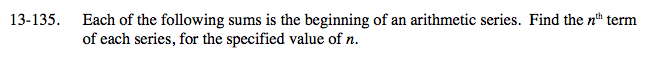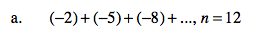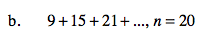### Home > A2C > Chapter 13 > Lesson 13.2.3 > Problem13-135

13-135.
1. Each of the following sums is the beginning of an arithmetic series. Find the nth term of each series, for the specified value of n. Homework Help ✎

1. (−2) + (−5) + (−8) + …, n = 12

2. 9 + 15 + 21 + …, n = 20Set up an equation to describe the sequence.

t(n) = −2 − 3(n − 1)

Now substitute 12 for n to find the 12th term.

t(12) = −2 − 3(11)

−35See part (a).